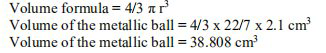# NCERT Solutions Class 9 Mathematics Solutions for Surface Areas and Volumes - Exercise 13.8 in Chapter 13 - Surface Areas and Volumes

The diameter of a metallic ball is 4.2 cm. What is the mass of the ball, if the density of the metal

is 8.9 g per cm3? (Assume π = 22/7)

diameter of a metallic ball = 4.2 cm

Radius(r) of the metallic ball, r = 4.2/2 cm = 2.1 cmNow, using relationship between, density, mass and volume,

Density = Mass/Volume

Mass = Density x volume

= (8.9 x 38.808) g

= 345.3912 g

The mass of the ball is 345.39 g (approx).

Related Questions

Lido

Courses

Teachers

Book a Demo with us

Syllabus

Maths
CBSE
Maths
ICSE
Science
CBSE

Science
ICSE
English
CBSE
English
ICSE
Coding

Terms & Policies

Selina Question Bank

Maths
Physics
Biology

Allied Question Bank

Chemistry
Connect with us on social media!